# E8 folding to H4+H4/φ

I found the rotation matrix that shows the E8 Dynkin diagram can indeed be folded to H4+H4/φ.

The H4 and its 120 vertices make up the 4D 600 Cell. It is made up of 96 vertices of the Snub 24-Cell and the 24 vertices of the 24-Cell=[16 vertex Tesseract=8-Cell and the 8 vertices of the 4-Orthoplex=16-Cell]).

It can be generated from the 240 split real even E8 vertices using a 4×8 rotation matrix:
x = (1, φ, 0, -1, φ, 0, 0, 0)
y = (φ, 0, 1, φ, 0, -1, 0, 0)
z = (0, 1, φ, 0, -1, φ, 0, 0)
w = (0, 0, 0, 0, 0, 0, φ^2, 1/φ)

where φ=Golden Ratio=(1+Sqrt(5))/2

It is also interesting to note that the x, y, and z vectors project to a hull of the 3D Rhombic Triacontrahedron from the 6D 6 cube Hexaract (which then generates the hull of the Dodecahedron and Icosahedron Platonic solids).

Here’s a look at the Dynkin Diagram folding of E8 to H4+H4/φ: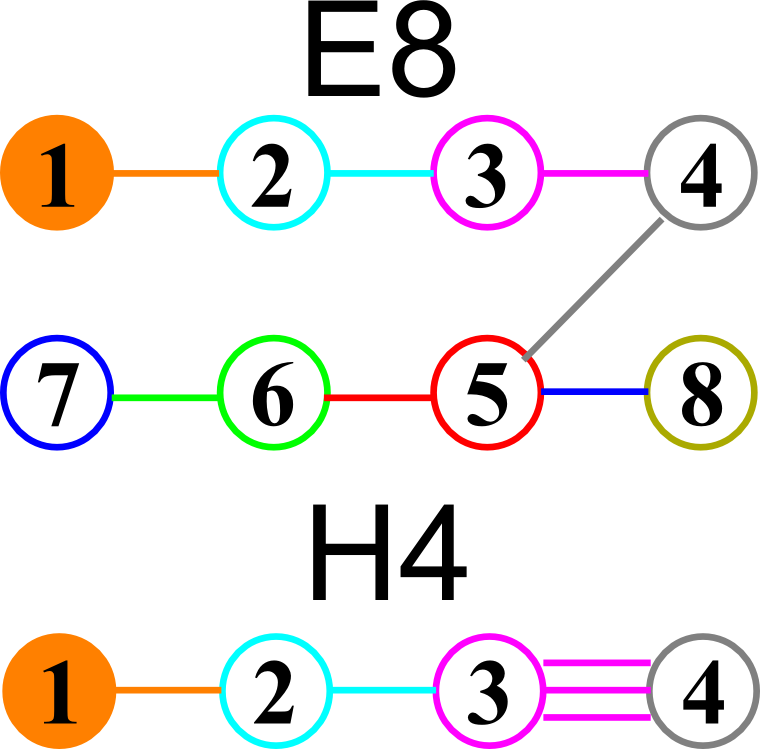I find in folding from 8D to 4D, that the 6720 edge counts split into two sets of 3360 from E8’s 6720 length Sqrt(2), but the combined edges and vertices recreate the E8 petrie diagram perfectly.

Some visualizations of this in 8D: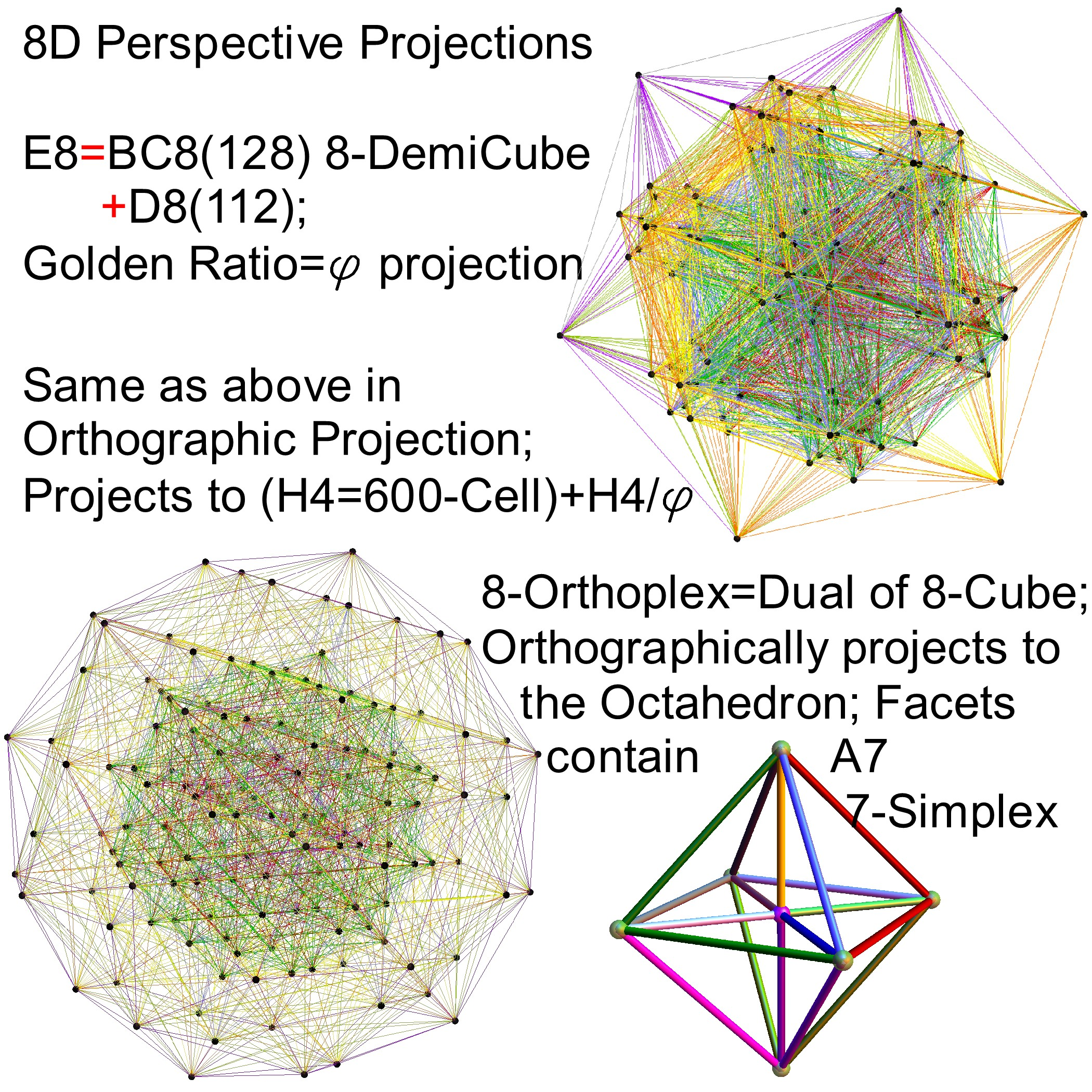and also showing the Rhombic Triacontrahedron folding from 6D:# Complete Integration of Octonions with E8

I’ve updated all .CDF, .NB and older .NBP demonstration code with Mathematica v.9

This now includes the completed integration of octonions with E8 & the extended Stadard Model particle assignments. This means the 480 octonions are in fact in a 2:1 cover of E8’s 240 vertices (with their association to Lisi’s particle assignments). This creates the opportunity for a self-dual type of “super symmetry” where all three generations emerge from the octonions.

# E8 to 4D (3D+T)

E8 8D to 4D (3D+T) Animation

Shown in this animation are the 240 vertices of E8 with shape, size, and color assigned based on theoretical physics of an extended Standard Model (eSM). It is made up of three sets of 120 frames, each with a different algorithm for calculating perspective and orthogonal, rotational and translational 8D flight paths. It is interesting to note that it is the 8D camera that is moving through 8D space and the vertices remain in their same 8D position.

The 30 blue triangles represent E8 triality relationships using an 8D rotation matrix based on 2Pi/3 (or 120 degrees). Each vertex in a blue triangle is transformed into an adjacent one by the dot product with the matrix. A second transformation transforms it to the next, while the third recovers the original vertex.

The 28 red and green triangles are created from a subset of the 6720 (shortest) edges of 8D norm’d length Sqrt(2). These are filtered to represent the particle sums (linked by a red line) for a common (clicked) vertex (linked by 2 green lines). It is interesting to note that all sums for a given vertex are only found in adjacent vertices.

Higher definition (2 sets 60 frames each):

# Dynkin demonstration published

After significant peer review, my Dynkin Diagram Visualizer was published at Wolfram Demonstrations. Please see http://demonstrations.wolfram.com/DynkinDiagrams/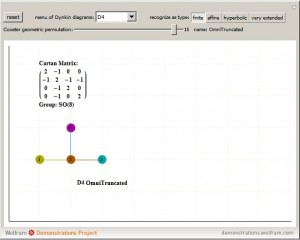# A Free Interactive Visual ToE Demonstration

This free Mathematica Computable Document Format (.CDF) demonstration takes you on an integrated visual journey from the abstract elements of geometry, algebra, particle and nuclear physics, and on to the atomic elements of chemistry. http://theoryofeverything.org/TOE/JGM/ToE_Demonstration.cdf (0.5Mb)
It requires the free Mathematica CDF plugin.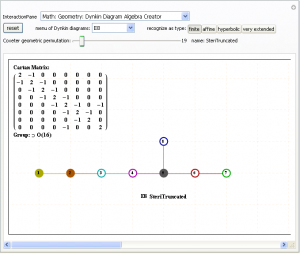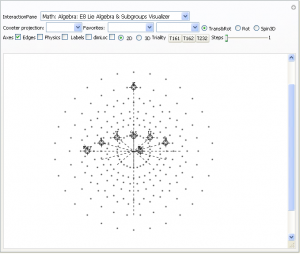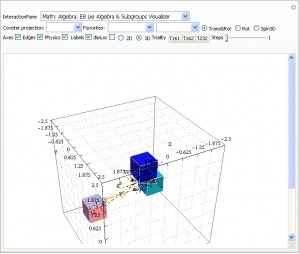# Ho-Mg-Zn QuasiCrystal Electron Diffraction with E8 5Cube Projection

This is an image of the electron diffraction pattern of an icosahedral Zn-Mg-Ho quasicrystal with an overlay of a 5-Cube projection from the 240 vertices of the split real even E8 Lie Group.

The basis vectors for the E8 projection are shown (1:1 with the ring of gray vertices with the last 3 of 8 dimensions 0).

There are 2480 overlapping edge lines from the 240 E8 vertices. They have norm’d unit length calculated from the 5 non-zero projected dimensions of E8. Of these, 32 inner vertices and 80 edges belong to the 5D 5-Cube (Penteract) proper. Edges are shown with colors assigned based on origin vertex distance from the outer perimeter.

The vertex colors of the 5-Cube projection represent E8 vertex overlaps. These are:
InView vertices={color{overlap,count},…}Total
{LightGreen{1,20},Pink{5,20},Gray{10,10},Orange{20,1}}51
All vertices={color{overlap,count},…}Total
{LightGreen{1,20},Pink{5,100},Gray{10,100},Orange{20,20}}240

A related projection of a 6D 6-Cube (Hexeract) into a perspective 3D object using the Golden Ratio [Phi]. This particular projection is used to understand the structure of QuasiCrystals. The specific basis vectors are:
x = {1, [Phi], 0, -1, [Phi], 0}
y = {[Phi], 0, 1, [Phi], 0, -1}
z = {0, 1, [Phi], 0, -1, [Phi]}
There are 64 vertices and 192 unit length edges forming pentagonal symmetry along specific axis (as well as hexagonal symmetries on other axis).